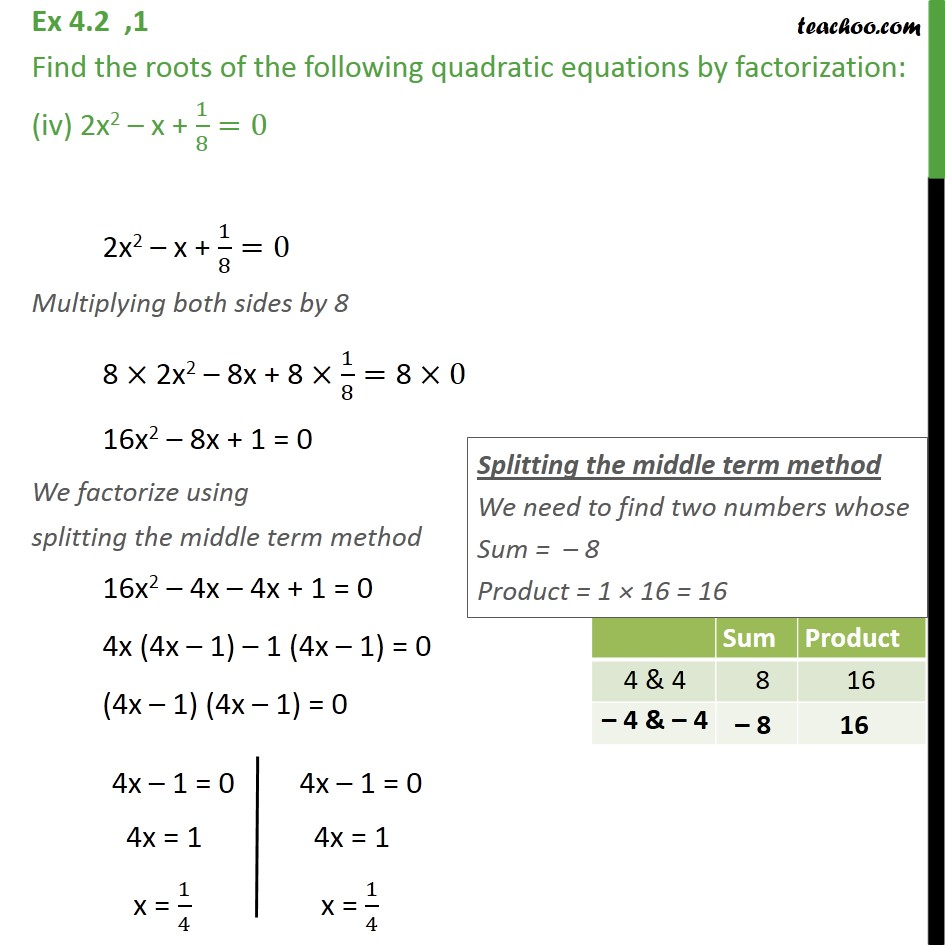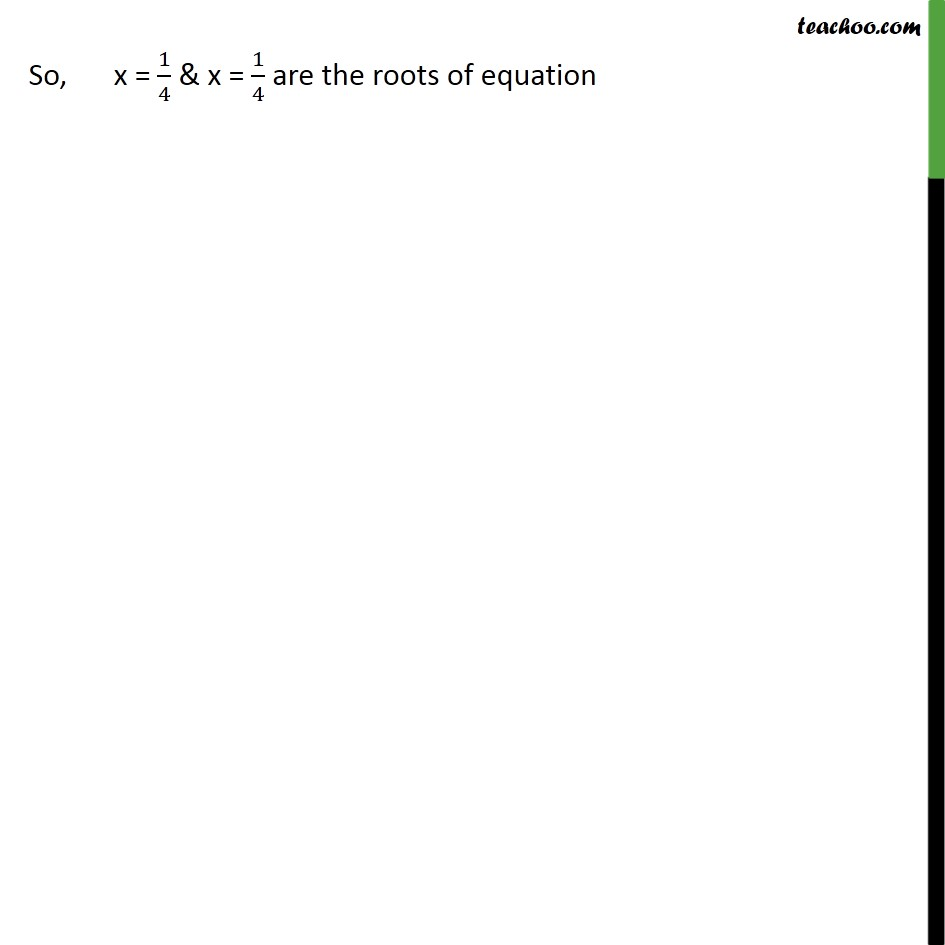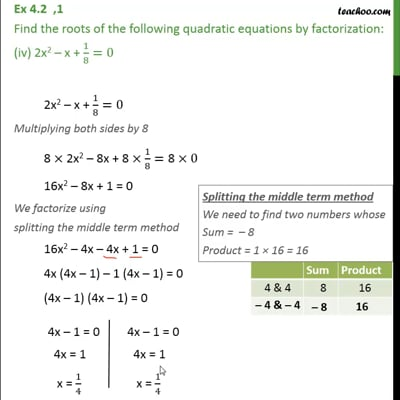Ex 4.2

Chapter 4 Class 10 Quadratic Equations
Serial order wiseThis video is only available for Teachoo black users

Introducing your new favourite teacher - Teachoo Black, at only ₹83 per month

### Transcript

Ex 4.2 ,1 Find the roots of the following quadratic equations by factorization: (iv) 2x2 x + 1/8=0 2x2 x + 1/8=0 Multiplying both sides by 8 8 2x2 8x + 8 1/8="8 " 0 16x2 8x + 1 = 0 We factorize using splitting the middle term method 16x2 4x 4x + 1 = 0 4x (4x 1) 1 (4x 1) = 0 (4x 1) (4x 1) = 0 So, x = 1/4 & x = 1/4 are the roots of equation